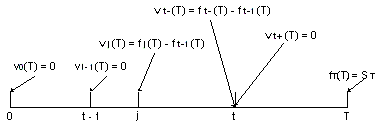### Why should I choose AnalystNotes?

AnalystNotes specializes in helping candidates pass. Period.

### Subject 1. Generic pricing and valuation of a futures contract

To avoid an arbitrage opportunity, the futures price must converge to the spot price at expiration: fT(T) = ST.

• fT(T): the price of a futures contract at time T that expires at time T.
• ST: the price of the underlying asset in the spot market at time T.

If this equation did not hold, what would happen?

• If fT(T) < ST, a trader would buy the futures contract, let it expire immediately, and pay fT(T) to get the underlying asset. Essentially he would have paid an amount smaller than the spot price to get an asset.
• If fT(T) > ST, a trader would simply do the opposite (sell a futures and buy the asset to capture an immediate profit at no risk).
• Only if fT(T) = ST does this arbitrage opportunity go away.

If expiration is right now, a futures transaction is equivalent to a spot transaction, so the futures price must equal the spot price.

Valuation of futures.

As no money changes hands, the value of a futures contract at the initiation is zero: v0(T) = 0.

Now let us determine the value of the contract during its life.Now suppose we are at the end of day t during the contract's life. This point would be at time t.

• t-: an instance before time t.
• t+: an instance after time t.
• In both cases the futures price is ft(T).

The value of a futures contract is:

• vt-(T) = ft(T) - ft-1(T): the contract was previously marketed to market at the end of day t - 1 to a price of ft-1(T).
• An instance later when the futures contract is marked to market, the trader will receive a gain of ft(T) - ft-1(T). We can reasonably ignore the present value difference of receiving this money an instance later.
• vt+(T) = 0 as soon as the account is marked to market.

Therefore, the value of a futures contract before it has been marked to market is the gain or loss accumulated since the account was last marked to market.

• The holder of a futures contract has a claim or liability on this account. Once that claim is captured or the liability paid through the mark-to-market process, the contract is repriced to its current market price and the claim or liability goes back to a value of zero.
• An instance before expiration, the value of a futures contract is simply the accumulated profit since the last mark to market.
• At expiration, the value goes back to zero.
• With respect to the value of the futures, expiration is no different from any other day.

Because a typical futures contract is generally actively traded, its value is simply the observable price change since the last mark to market.

#### Practice Question 1

The value of a futures contract is:

A. accumulated gain or loss since the last mark to market.
B. the observable futures price in the market.
C. the difference between the current price of the futures and the price when the futures contract was created.

The value of a futures contract before it has been marked to market is the gain or loss accumulated since the amount was last marked to market.

#### Practice Question 2

As soon as a futures contract is marked to market, the price of the contract is

A. the current spot price of the underlying.
B. accumulated gain or loss since the last mark to market.
C. the observable futures price in the market.